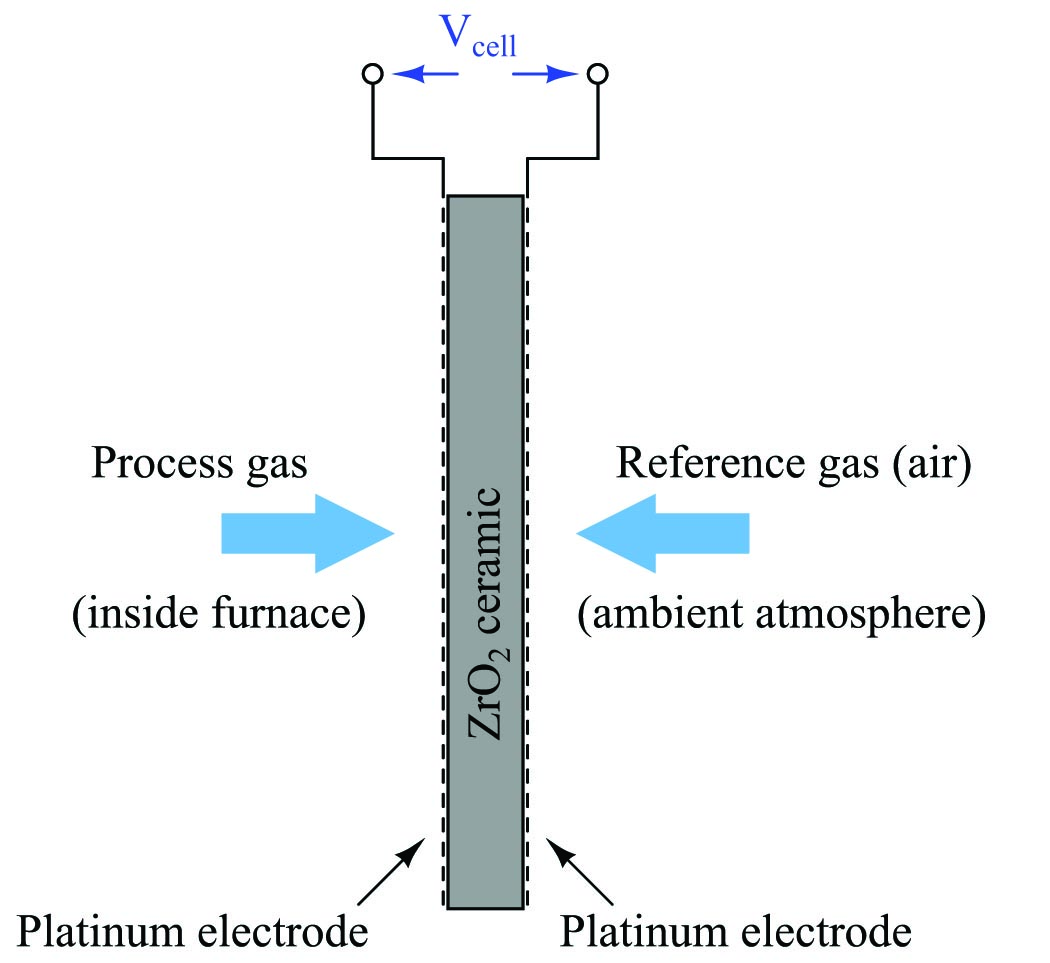Analytical Measurements of Chemical Compositions

Chapter 26 - Signal Characterization in Control Systems

A great many chemical composition measurements may be made indirectly by means of electricity, if those measurements are related to the concentration of ions (electrically charged molecules). Such measurements include:

• pH of an aqueous solution
• Oxygen concentration in air
• Ammonia concentration in air

The basic principle works like this: two different chemical samples are placed in close proximity to each other, separated only by an ion-selective membrane able to pass the ion of interest. As the ion activity attempts to reach equilibrium through the membrane, an electrical voltage is produced across that membrane. If we measure the voltage produced, we can infer the relative activity of the ions on either side of the membrane.

Not surprisingly, the function relating ion activity to the voltage generated is nonlinear. The standard equation describing the relationship between ionic activity on both sides of the membrane and the voltage produced is called the Nernst equation:

$V = {{R T} \over {nF}} \ln \left({a_1 \over a_2}\right)$

Where,

$$V$$ = Electrical voltage produced across membrane due to ion exchange (volts)

$$R$$ = Universal gas constant (8.315 J/mol$$\cdot$$K)

$$T$$ = Absolute temperature (Kelvin)

$$n$$ = Number of electrons transferred per ion exchanged (unitless)

$$F$$ = Faraday constant (96485 coulombs per mole)

$$a_1$$ = Activity of ion in measured sample

$$a_2$$ = Activity of ion in reference sample (on other side of membrane)

A practical application for this technology is in the measurement of oxygen concentration in the flue gas of a large industrial burner, such as what might be used to heat up water to generate steam. The measurement of oxygen concentration in the exhaust of a combustion heater (or boiler) is very important both for maximizing fuel efficiency and for minimizing pollution (specifically, the production of NO$$_{x}$$ molecules). Ideally, a burner’s exhaust gas will contain no oxygen, having consumed it all in the process of combustion with a perfect stoichiometric mix of fuel and air. In practice, the exhaust gas of an efficiently-controlled burner will be somewhere near 2%, as opposed to the normal 21% of ambient air.

One way to measure the oxygen content of hot exhaust is to use a high-temperature zirconium oxide detector. This detector is made of a “sandwich” of platinum electrodes on either side of a solid zirconium oxide electrolyte. One side of this electrochemical cell is exposed to the exhaust gas (process), while the other side is exposed to heated air which serves as a reference:The electrical voltage generated by this “sandwich” of zirconium and platinum is sent to an electronic amplifier circuit, and then to a microcomputer which applies an inverse function to the measured voltage in order to arrive at an inferred measurement for oxygen concentration. This type of chemical analysis is called potentiometric, since it measures (“metric”) based on an electrical voltage (“potential”).

The Nernst equation is an interesting one to unravel, to solve for ion activity in the sample ($$a_1$$) given voltage ($$V$$):

$V = {{R T} \over {nF}} \ln \left({a_1 \over a_2}\right)$

$\hbox\textit{Multiplying both sides by } nF \hbox{:}$

$nFV = {R T} \ln \left({a_1 \over a_2}\right)$

$\hbox\textit{Dividing both sides by } RT \hbox{:}$

${nFV \over RT} = \ln \left({a_1 \over a_2}\right)$

$\hbox\textit{Applying the rule that the difference of logs is equal to the log of the quotient:}$

${nFV \over RT} = \ln a_1 - \ln a_2$

$\hbox\textit{Adding } \ln a_2 \hbox{ to both sides:}$

${nFV \over RT} + \ln a_2 = \ln a_1$

$\hbox\textit{Making both sides of the equation a power of } e \hbox{:}$

$e^{{nFV \over RT} + \ln a_2} = e^{\ln a_1}$

$\hbox\textit{Canceling the natural log and exponential functions on the right-hand side:}$

$e^{{nFV \over RT} + \ln a_2} = a_1$

In most cases, the ionic activity of $$a_2$$ will be relatively constant, and so $$\ln a_2$$ will be relatively constant as well. With this in mind, we may simplify the equation further, using $$k$$ as our constant value:

$\hbox\textit{Substituting } k \hbox{ for } \ln a_2 \hbox{:}$

$e^{{nFV \over RT} + k} = a_1$

$\hbox\textit{Applying the rule that the sum of exponents is the product of powers:}$

$e^k e^{nFV \over RT} = a_1$

$\hbox{If } k \hbox{ is constant, then } e^k \hbox{ will be constant as well (calling the new constant } C \hbox{):}$

$C e^{nFV \over RT} = a_1$

Analytical instruments based on potentiometry must evaluate this inverse function to “undo” the Nernst equation to arrive at an inferred measurement of ion activity in the sample given the small voltage produced by the sensing membrane. These instruments typically have temperature sensors as well built in to the sensing membrane assembly, since it is apparent that temperature ($$T$$) also plays a role in the generation of this voltage. Once again, this mathematical function is typically evaluated in a microprocessor.

Published under the terms and conditions of the Creative Commons Attribution 4.0 International Public License